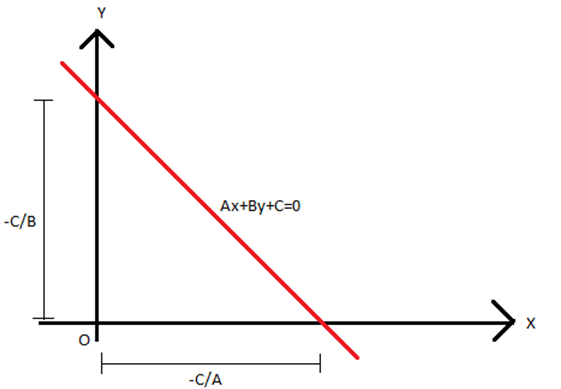# Equation of a Line

The standard forms of the equation of a line are:

• Slope-intercept form
• Intercept form
• Normal form

Let us learn all the straight lines formulas along with the general equation of a line and different forms to find the equation of a straight line in detail here.

## General Equation of a Line

The general equation of a line in two variables of the first degree is represented as

Ax + By +C = 0,

A, B ≠ 0 where A, B and C are constants which belong to real numbers.

When we represent the equation geometrically, we always get a straight line.

Below is a representation of straight-line formulas in different forms:

## Slope-intercept Form

We know that the equation of a straight line in slope-intercept form is given as:

 y = mx + c

Where m indicates the slope of the line and c is the y-intercept
When B ≠ 0 then, the standard equation of first degree Ax + By + C = 0 can be rewritten in slope-intercept form as:

y = (− A/B) x − (C/B)

Thus, m= –A/B and c = –C/B .

## Intercept Form

The intercept of a line is the point through which the line crosses the x-axis or y-axis. Suppose a line cuts the x-axis and y-axis at (a, 0) and (0, b), respectively. Then, the equation of a line making intercepts equal to a and b on the x-axis and the y-axis respectively is given by:

x/a + y/b = 1

Now in case of the general form of the equation of the straight line, i.e. Ax+By+C = 0, if C ≠ 0, then Ax + By + C = 0 can be written as;

x/(-C/A) + y/(-C/B) = 1

where a = -C/A and b = – C/B## Normal Form

The equation of the line whose length of the perpendicular from the origin is p and the angle made by the perpendicular with the positive x-axis is given by α is given by:

x cos α+y sin α = p

This is known as the normal form of the line.

In case of the general form of the line Ax + By + C = 0 can be represented in normal form as:

A cos α = B sin α = – p

From this we can say that cos α = -p/A and sin α = -p/B.
Also it can be inferred that,

cos2α + sin2α = (p/A)2 + (p/B)2

1 = p2 (A2 + B2/A2 .B2)

$$\begin{array}{l}\Rightarrow p = \left (\frac{AB}{\sqrt {A^{2} + B^{2}}} \right )\end{array}$$

From the general equation of a straight line Ax + By + C = 0, we can conclude the following:

• The slope is given by -A/B, given that B ≠ 0.
• The x-intercept is given by -C/A and the y-intercept is given by -C/B.
•  It can be seen from the above discussion that:
$$\begin{array}{l}p = \pm\left (\frac{AB}{\sqrt {A^{2} + B^{2}}} \right )\end{array}$$
,
$$\begin{array}{l}\cos \alpha = \pm \left (\frac{B}{\sqrt {A^{2} + B^{2}}} \right )\end{array}$$
,
$$\begin{array}{l}\sin \alpha = \pm \left (\frac{A}{\sqrt {A^{2} + B^{2}}} \right )\end{array}$$
• If two points (x1, y1) and(x2, y2)are said to lie on the same side of the line Ax + By + C = 0, then the expressions Ax1+ By1 + C  and Ax2 + By2 + C will have the same sign or else these points would lie on the opposite sides of the line.

## Straight Line Formulas

Let us accumulate the straight line formulas we have discussed so far:

 Slope (m) of a non-vertical line passing through the points (x1 , y1 ) and (x2, y2 ) m=(y2-y1)/(x2-x1), x1≠x2 Equation of a horizontal line y = a or y=-a Equation of a vertical line x=b or x=-b Equation of the line passing through the points (x1 , y1 ) and (x2, y2 ) y-y1= [(y2-y1)/(x2-x1)]×(x-x1) Equation of line with slope m and intercept c y = mx+c Equation of line with slope m makes x-intercept d. y = m (x – d). Intercept form of the equation of a line (x/a)+(y/b)=1 The normal form of the equation of a line x cos α+y sin α = p

### Example of Straight Lines

To understand this concept better go through the below examples:

(1) The equation of a line is given by, 2x – 6y +3 = 0. Find the slope and both the intercepts.
Solution:

The given equation 2x – 6y + 3 = 0 can be represented in slope-intercept form as:

y = x/3 + 1/2

Comparing it with y = mx + c,
Slope of the line, m = 1/3

Also, the above equation can be re-framed in intercept form as;

x/a + y/b = 1

2x – 6y = -3

x/(-3/2) – y/(-1/2) = 1

Thus, x-intercept is given as a = -3/2 and y-intercept as b = 1/2.

(2) The equation of a line is given by, 13x – y + 12 = 0. Find the slope and both the intercepts.

Solution: The given equation 13x – y + 12 = 0 can be represented in slope-intercept form as:

y = 13x + 12
Comparing it with y = mx + c,
Slope of the line, m = 13

Also, the above equation can be re-framed in intercept form as;

x/a + y/b = 1

13x – y = -12

x/(-12/13) + y/12 = 0

Thus, x-intercept is given as a = -12/13 and y-intercept as b = 12.

To learn all the concepts in Math in a more engaging way, register at BYJU’S. Also, watch interesting videos on various maths topics by downloading BYJU’S– The Learning App from Google Play Store or the app store.